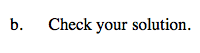### Home > CAAC > Chapter 4 > Lesson 4.1.2 > Problem4-13

4-13.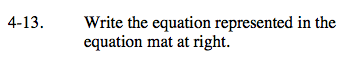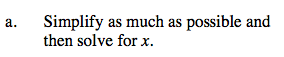Remove zero pairs.

Combine like terms.

Subtract x from both sides.

Divide by 2 on both sides.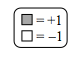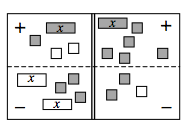Right now the equation is:

x + 1 + −2 −(−2x+3) = x + 5 −(2 + −1)

x +1 + (−2) + 2x + (−3) = x +5 + −2 + 1

3x + (−4) = x + 4

3x = x + 8

2x = 8

x = 4